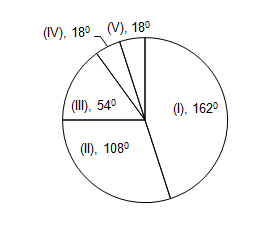# Data Interpretation - Pie charts

Basics:
Total angle at the center of a pie chart = ${360^0}$
To convert k% percentage into angle = $\displaystyle\frac{k}{{100}} \times {360^0}$
To convert ${m^0}$ into percentage = $\displaystyle\frac{m}{{360}} \times 100$

Set 1 : The various sections of the population are indicated below in the pie-chart. Study the pie-chart and answer the following questions:
The total population of a city is 5000I.  Employees of the Public Sector II. Employees of the Private Sector
III. Employees of the Corporate Sector IV. Self-Employed   V. Unemployed.

1. What percentage of the employed persons is self employed?
 a.   5% b.  5 5/19 % c.  19% d.  20%
Solution:  Total Employed = Total population - Unemployed = ${360^0} - {18^0} = {342^0}$
Now self employed are ${18^0}$. So self employed as a percentage of employed = $\displaystyle\frac{{18}}{{342}} \times 100 = 5\displaystyle\frac{5}{{19}}\%$

2. Number of persons employed in the Corporate Sector is
 a.   250 b.  500 c.  750 d.  1500
Solution: We have to convert degrees into numbers.  So $\displaystyle\frac{{54}}{{360}} \times 5000 = 750$

3. The number of Unemployed persons is
 a.   250 b.  150 c.  100 d.  50
Solution: We have to convert degrees into numbers. So $\displaystyle\frac{{18}}{{360}} \times 5000 = 250$
Shortcut: We calculated corporate sector employees as 750. But from pie chart Corporate sector employees are 3 times of unemployed. So 1/3rd of 750 = 250

4. The number of persons employed in both the Public Sector and Corporate Sector is
 a.   3750 b.  3000 c.  2500 d.  2200
Solution: Number of persons employed in public sector and corporate sector together = 54 + 162 = 216.
$\displaystyle\frac{{54 + 162}}{{360}} \times 5000 = \displaystyle\frac{{216}}{{360}} \times 5000 = 3000$

5. What percentage of the employed persons is employed in Private Sector ?
 a.   29% b.  31 11/19% c.  34% d.  31%
From the 1st question, employed are 342. and From pie chart private sector employees are 108.
$\displaystyle\frac{{108}}{{342}} \times 100 = \displaystyle\frac{{600}}{{19}} = 31\frac{{11}}{{19}}\%$

Set 2:  These questions are based on following graphs Classification of appeared candidates in a competitive test from different states and qualified candidates from those states.

1. What is the ratio between the number of appeared candidates from states C and E together and the appeared candidates from states A and F together ?
 a.   17 : 33 b.  11 : 13 c.  13 : 27 d.  17 : 27
Solution: There is not need to calculate values. This is simply a ratio. So we can compare the ratio of their percentages. C + E = 8 + 9 = 17; A + F = 15 + 18 = 33.
So ratio = 17 : 33
2. In which State the percentage of qualified candidates to that of appeared candidates is minimum ?
 a.  C b.  F c.  D d.  E
Solution:  This is a lengthy question. But we will use simple technique to solve this question.
We have to calculate the ratio of Qualified to appeared for each state. So $\dfrac{{{\text{Qualified from A}}}}{{{\text{Selected from A}}}}{\rm{ \times 100}}$ = $\dfrac{{18\% \times 9000}}{{15\% \times 45000}} \times 100$
But If you observe in the above equation, only 18% / 15% changes for each state. Remaining values are constant. Minimum percentage we get if numerator is small and denominator is big.
For C it is 7/8 = 1/1.14 and for E it is 9/14 = 1/1.5 So for E denominator is big. So it has the ratio Minimum.
So Option D is correct.

3. What is the difference between the number of qualified candidates of states D and G
 a.   690 b.  670 c.  780 d.  720
Solution: Instead of calculating qualified candidates for D and G separately, we take the difference in their percentages.  i.e., 8% (9000) = 720.

4. What is the percentage of qualified candidates to that of appeared candidates from states B and C taken together ?
 a.   23.11 b.  24.21 c.  21.24 d.  23
Qualified candidates from B and C = (16 + 7)% = 23% (9000)
Appeared candidates from B and C = (11 + 8)% = 19% (45000)
So required percentage = $\displaystyle\frac{{23\% (9000)}}{{19\% (45000)}} \times 100 = \displaystyle\frac{{23}}{{19 \times 5}} \times 100 = 24.21$

5. What is the ratio between number of candidates qualified from states B and D together and the number of candidates appeared from state C respectively ?
 a.   8 : 37 b.  11 : 12 c.  37 : 48 d.  7 : 37
Solution: The required ration = (16 + 21)% (9000) : 8% (45000) $\Rightarrow$ 37 : 8 x 5 $\Rightarrow$ 37 : 40